# How To Compare Format Of Two Cells In Microsoft Excel

In this article, you will learn how to compare format of two cells.

Example(1):TODAY function entered in cell A2 & B2 shows the same date with different format.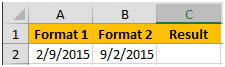Format of cell A2 is below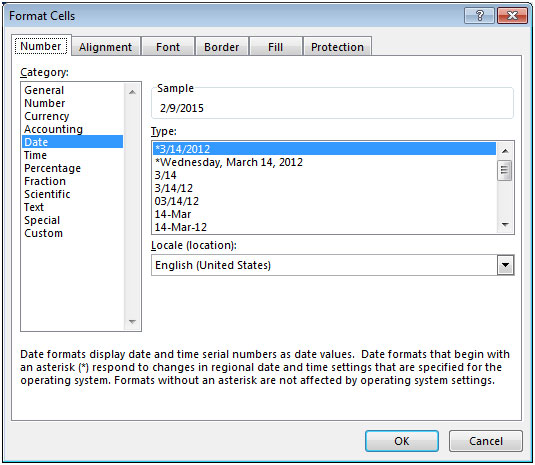Format of cell A3 is below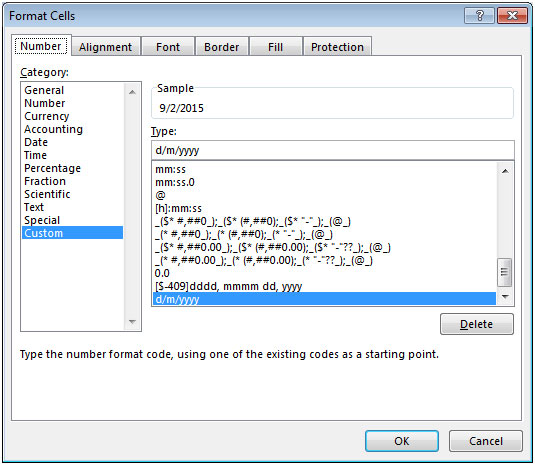In the below snapshot, we would like to see the result as FALSE since the formats are different from each other.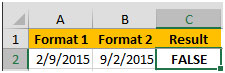Example (2): cell A3 & B3 contain 10.11 as a number with different formatting.

Cell A3 has the Number format with only 1 decimal places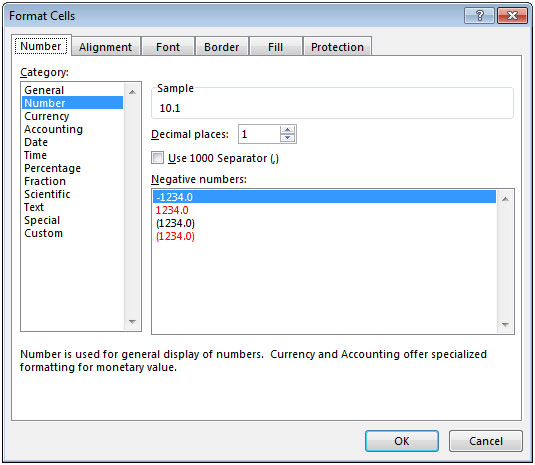Cell B3 has the Number format with only 2 decimal places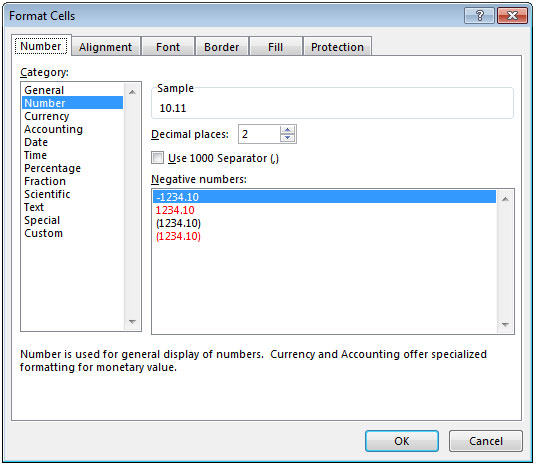Hence, both the cells contain the same value with different format. Here, we would like to see the result as FALSE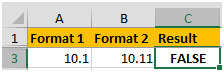Example (3): Cell A4 contains 20.10 & cell B4 contains 20.11, even though the values are different in both the cells but they have similar format i.e. Number format with showing 2 decimal places.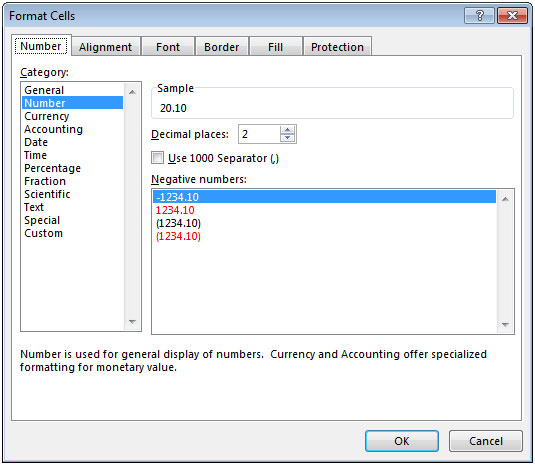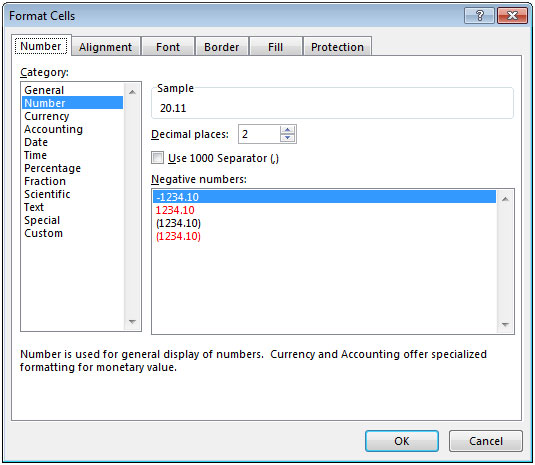Here, we would like to see the result as TRUE because of the similar formats.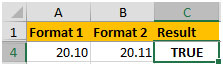We will use CELL function to get the output.

In cell C2, the formula would be =(CELL("format",A2))=(CELL("format",B2))

After copying down the formula, we will get the desired result.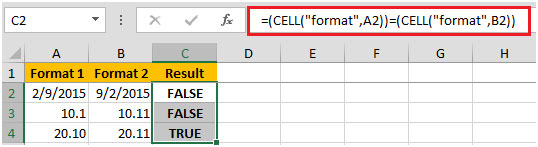In this way, we can compare the formats of two cells.

1.2.